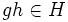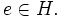# Intersection of subgroups is subgroup

(diff) ← Older revision | Latest revision (diff) | Newer revision → (diff)
Jump to: navigation, search
This article gives the statement, and possibly proof, of a basic fact in group theory.
View a complete list of basic facts in group theory
VIEW FACTS USING THIS: directly | directly or indirectly, upto two steps | directly or indirectly, upto three steps|
VIEW: Survey articles about this

## Statement

### Verbal statement

The intersection of any arbitrary collection of subgroups of a group is again a subgroup.

### Symbolic statement

Let$H_i\!$ be an arbitrary collection of subgroups of a group$G\!$ indexed by$i \in I$. Then,$\textstyle\bigcap_{i \in I} H_i$ is again a subgroup of$G\!$.

Note that if the collection$I\!$ is empty, the intersection is defined to be the whole group. In this case, the intersection is clearly a subgroup. It should be noted that the case of an empty intersection is covered in the language of the general proof.

## Related facts

For examples, see the article Intersection of subgroups

### The related notion of join of subgroups

Given a collection of subgroups, their join is defined as the smallest subgroup containing all of them; equivalently, it is the intersection of all subgroups containing them.

This is closely related to the notion of the subgroup generated by a subset. The subgroup generated by a subset is the intersection of all subgroups containing that subset.

Notice that although the union of subgroups is not a subgroup, the fact that an intersection of subgroups is a subgroup tells us that there is a smallest subgroup containing any given collection of subgroups. This is analogous to the fact that the greatest lower bound property on a totally ordered set yields the least upper bound property.

### Other facts about intersections of subgroups

A subgroup property is termed:

• intersection-closed if the intersection of an arbitrary nonempty collection of subgroups with the property also has the property.
• finite-intersection-closed if the intersection of a finite nonempty collection of subgroups with the property also has the property.
• strongly intersection-closed if it is intersection-closed and also true for the whole group as a subgroup of itself. Thus, it is preserved on taking intersections of possibly empty collections.
• strongly finite-intersection-closed if it is finite-intersection-closed and also true for the whole group as a subgroup of itself.

There are some basic results of importance about intersection-closedness:

### Intersections of subsets other than subgroups

Subset property Proof that it is closed under arbitrary intersections
twisted subgroup intersection of twisted subgroups is twisted subgroup
1-closed subset intersection of 1-closed subsets is 1-closed subset
symmetric subset intersection of symmetric subsets is symmetric subset

### Analogues in other algebraic structures

For any variety of algebras, an intersection of subalgebras is a subalgebra. The proof is exactly the same as that for groups. In fact, the result holds in a slightly greater generality than varieties of algebras. For instance, an intersection of subfields is a subfield, although fields do not form a variety of algebras.

## Proof

Given: Let$H_i\!$ be an arbitrary collection of subgroups of a group$G\!$ indexed by$i \in I.$ Let us denote$H = \textstyle\bigcap_{i \in I} H_i.$ Here,$e\!$ denotes the identity element of$G.\!$

To prove: We need to show that$H$ is a subgroup. In other words, we need to show the following:

1.$e \in H$
2. If$g \in H$ then$g^{-1} \in H$
3. If$g, h \in H$ then$gh \in H$

Proof: Let's prove these one by one:

1. Since$e \in H_i$ for every$i,\!$$e \in H.$
2. Take$g \in H$. Then$g \in H_i$ for every$i \in I.$ Since each$H_i\!$ is a subgroup,$g^{-1} \in H_i$ for each$i \in I.$ Thus,$g^{-1} \in H.$
3. Take$g, h \in H.$ Then$g, h \in H_i$ for every$i,\!$ so$gh \in H_i$ for every$i \in I.$ Thus$gh \in H.$